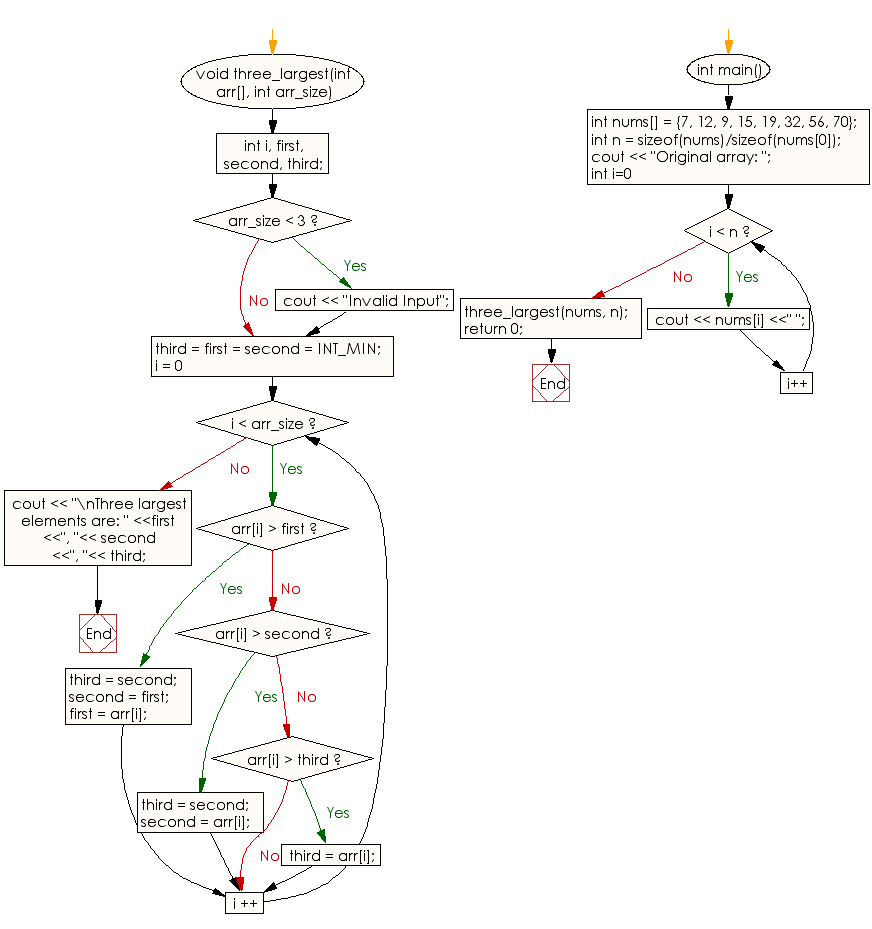﻿ C++ Exercises: Find the largest three elements in an array - w3resource# C++ Exercises: Find the largest three elements in an array

## C++ Array: Exercise-2 with Solution

Write a C++ program to find the largest three elements in an array.

Sample Solution:

C++ Code :

``````#include<iostream>
using namespace std;

void three_largest(int arr[], int arr_size)
{
int i, first, second, third;

if (arr_size < 3)
{
cout << "Invalid Input";
}

third = first = second = INT_MIN;
for (i = 0; i < arr_size ; i ++)
{
if (arr[i] > first)
{
third = second;
second = first;
first = arr[i];
}
else if (arr[i] > second)
{
third = second;
second = arr[i];
}

else if (arr[i] > third)
third = arr[i];
}

cout << "\nThree largest elements are: " <<first <<", "<< second <<", "<< third;
}
int main()
{
int nums[] = {7, 12, 9, 15, 19, 32, 56, 70};
int n = sizeof(nums)/sizeof(nums);
cout << "Original array: ";
for (int i=0; i < n; i++)
cout << nums[i] <<" ";
three_largest(nums, n);
return 0;
}
``````

Sample Output:

```Original array: 7 12 9 15 19 32 56 70
Three largest elements are: 70, 56, 32
```

Flowchart:C++ Code Editor:

What is the difficulty level of this exercise?

Test your Programming skills with w3resource's quiz.

﻿